GFG App
Open AppBrowser
Continue

# How to Create Automatically Extended Plot Ranges in Excel?

We can easily create charts with the help of data points and data sources. But, If we have more data points added to our data source we need our chart will get updated depending upon our data source. For example, if we want to plot a sales chart on monthly basis, we need to update our chart every time we will need to add a new month’s data. To overcome this problem, we will use automatically extending plot range. The dynamic extended plot range is a range that updates automatically when we add more data to it. And, Excel will update the chart depending on new data points added or deleted to the range dataset.

## Steps to Create Automatically Extended Plot Ranges in Excel

In this example, we will be using random monthly sales data and will create a dynamically extending plot range for that dataset. Below are the steps to Create Automatically Extended Plot Ranges in Excel,

Step 1: Create a Dataset. In this step, we will create our dataset. Here, we will use the following data as our dataset.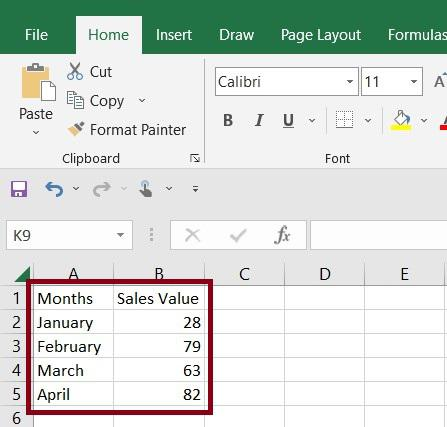Step 2: Create a Table. In this step, we will convert our dataset into a table. For this Select Data > Insert > Table.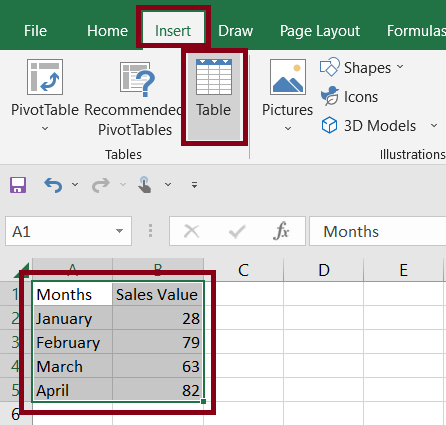Once, we click on Table, Excel will pop up a window about Create Table, we need to press the OK button.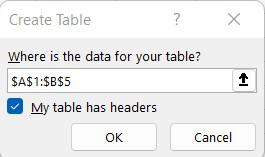After we click OK, Excel will convert our dataset into the table.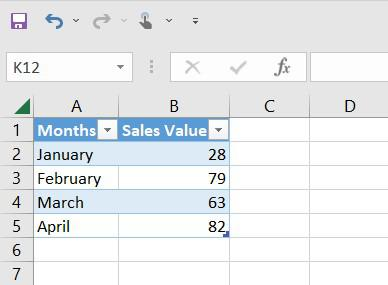Step 3: Insert Line Chart. In this step, we will insert a line chart for our table. For this Select Table Data > Insert > Charts > Line With Markers.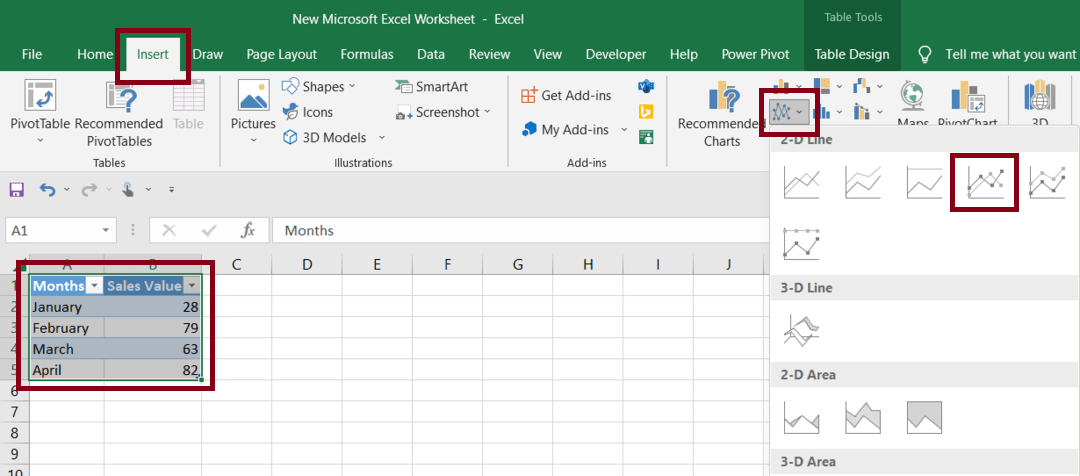Once, we click on Line With Markers, Excel will insert a line chart for our table data.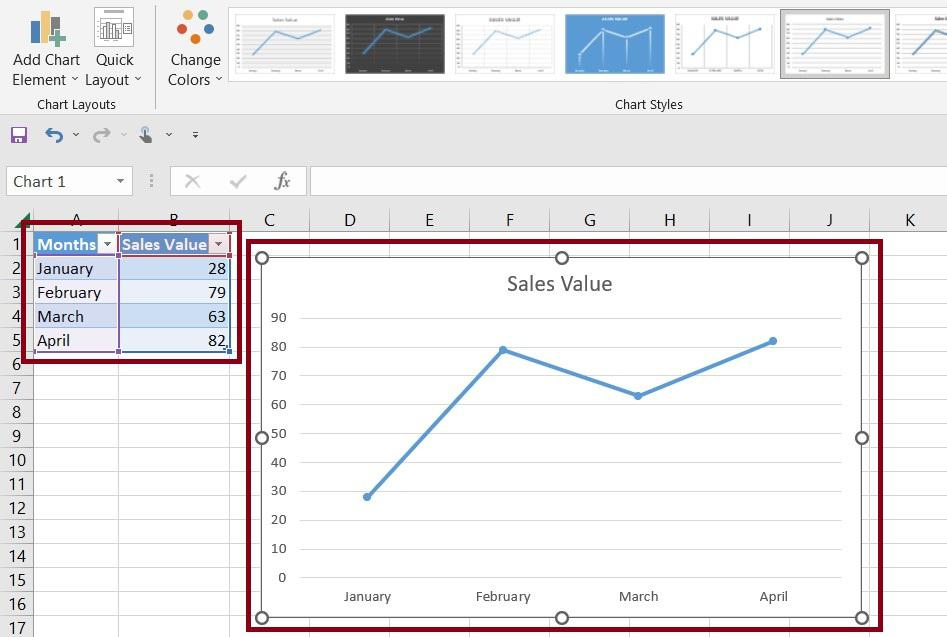Step 4: Adding More Range. Once, we have a map linked to our table, we can easily use excel table properties to create dynamically extending plot ranges. We need to hold the table in the bottom right corner and drag it down to create more range and add data points to it. Excel will automatically create the dynamically extending plot range.

My Personal Notes arrow_drop_up